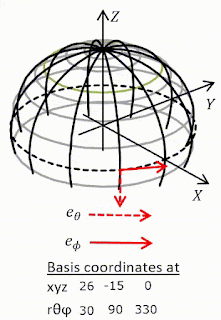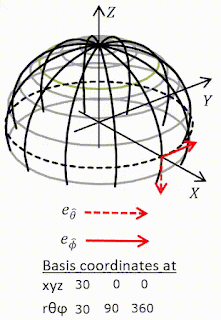## Thursday, 9 September 2021

### MTW Ex 8.1 Practice with tensor algebra

#### Question

Let $t,x,y,z$ be Lorentz coordinates in flat spacetime, and let $$r=\left(x^2+y^2+z^2\right)^\frac{1}{2}\ ,\ \theta=\arccos{\left(\frac{z}{r}\right)}\ ,\ \phi=\arctan{\left(\frac{y}{x}\right)}$$be the corresponding spherical coordinates. Then$$e_0=\frac{\partial\mathcal{P}}{\partial t}\ ,\ e_r=\frac{\partial\mathcal{P}}{\partial r}\ ,\ e_\theta=\frac{\partial\mathcal{P}}{\partial\theta}\ ,\ e_\phi=\frac{\partial\mathcal{P}}{\partial\phi}$$is a coordinate basis, and $$e_{\hat{0}}=\frac{\partial\mathcal{P}}{\partial t}\ ,\ e_{\hat{r}}=\frac{\partial\mathcal{P}}{\partial r}\ ,\ e_{\hat{\theta}}=\frac{1}{r}\frac{\partial\mathcal{P}}{\partial\theta}\ ,\ e_{\hat{\phi}}=\frac{1}{r\sin{\theta}}\frac{\partial\mathcal{P}}{\partial\phi}$$is a non-coordinate basis.
(a) Draw a picture of $e_\theta,e_\phi,e_{\hat{\theta}},e_{\hat{\phi}}$ at several different points on a sphere of constant $t,r$.
(b) What are the one-form bases dual to these tangent-vector bases?
(c) What is the transformation matrix linking the original Lorentz frame to the spherical coordinate frame $\left\{e_a\right\}$?
(d) Use this transformation matrix to calculate the metric components $g_{\alpha\beta}$ in the spherical coordinate basis and invert the result to get $g^{\alpha\beta}$.
(e) Show that the non-coordinate basis $\left\{e_{\hat{a}}\right\}$ is orthonormal everywhere; i.e. that $g_{\hat{\alpha}\hat{\beta}}=\eta_{\alpha\beta}$; i.e. that $$g=-\omega^{\hat{0}}\otimes\omega^{\hat{0}}+\omega^{\hat{r}}\otimes\omega^{\hat{r}}+\omega^{\hat{\theta}}\otimes\omega^{\hat{\theta}}+\omega^{\hat{\phi}}\otimes\omega^{\hat{\phi}}$$(f) Write the gradient of a function $f$ in terms of the spherical coordinate and noncoordinate bases.
(g) What are the components of the Levi-Civita tensor in the spherical coordinate and noncoordinate bases?

This exercise seems mainly to be concerned with putting the rules in Box 8.4 into practice. We meet a noncoordinate (anholonomic) basis. One of the features of these is that coordinates cannot be used to describe positions but you can use components to describe other tensors. So$$p^{\hat{\mu}}=\left(a,b,c,d\right)\equiv ae^{\hat{0}}+be^{\hat{r}}+ce^{\hat{\theta}}+de^{\hat{\phi}}$$is at best meaningless but$$g_{\hat{\alpha}\hat{\beta}}=\left(\begin{matrix}a&0&0&0\\0&b&0&0\\0&0&c&0\\0&0&0&d\\\end{matrix}\right)$$is valid and is the answer to (d) when $a=-1,b=c=d=1$ .
I found an excellent new way of  creating animated gifs. Which I used for the answer to (a) below. The diagrams for $e_{\hat{\theta}},e_{\hat{\phi}}$ are a good indication of (e).
 Coordinate bases going round equatorCoordinate bases going over poleNon-coordinate bases going round equatorNon-coordinate bases going round equator